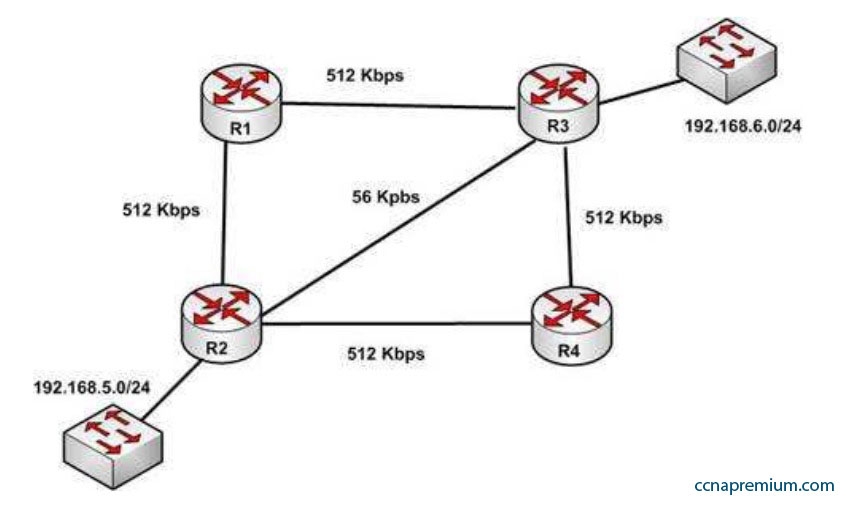# CISCO CCNA 200-301 Q25

With respect to the network shown below, which of the following statements are true when R2 sends a packet to the 192.168.6.0/24 network?A. If RIPv1 is in use, the path taken will be R2 – R4 – R3
B. If both RIPv2 and EIGRP are in use, the EIGRP route will be placed in the routing table
C. If EIGRP is in use, the only path taken will be R2 – R4 – R3
D. If RIPv2 is in use, the path taken will be R2 – R3

Explanation:
If both RIPv2 and EIGRP are in use, the EIGRP route will be placed in the routing table. If RIPv2 is in use, the path taken will be R2 – R3.

EIGRP has a default administrative distance (AD) of 90, while RIPv2 has a default administrative distance (AD) of 120. The route learned by the routing protocol with the lowest AD will be placed in the routing table.

If you wanted to force R2 to use the RIPv2 route instead of the EIGRP route, this could be accomplished by changing the administrative distance of RIPv2 to a value less than 90, such as 80. The commands that would accomplish this are:

R2(config)# router rip
R2(config-router)# distance 80

If either of the versions of RIP is in use, hop count is used to determine the route. The path with the least number of hops is R2 – R3. If RIPv1 is in use, the path taken would be R2 – R3, not R2 – R4 – R3, because R2 – R3 has a lower hop count.

If EIGRP is in use, the path R2 – R4 – R3 will not be the only path taken. EIGRP load-balances two equal cost paths when they exist, and R2 – R4 – R3 and R2 – R1 – R3 are of equal cost so would both be used.